# KSEEB SSLC Class 10 Maths Solutions Chapter 10 Quadratic Equations Ex 10.3

In this chapter, we provide KSEEB SSLC Class 10 Maths Solutions Solutions Chapter 10 Quadratic Equations Ex 10.3 for English medium students, Which will very helpful for every student in their exams. Students can download the latest KSEEB SSLC Class 10 Maths Solutions Solutions Chapter 10 Quadratic Equations Ex 10.3 pdf, free KSEEB SSLC Class 10 Maths Solutions Solutions Chapter 10 Quadratic Equations Ex 10.3 pdf download. Now you will get step by step solution to each question.

### Karnataka State Syllabus Class 10 Maths SolutionsChapter 10 Quadratic Equations Ex 10.3

Exercise 10.3 Class 10 Quadratic Equations Question 1.
Find the roots of the following quadratic equations, if they exist, by the method of completing the square:
(i) 2x2 – 7x + 3 = 0.
(ii) 2x2 + x – 4 = 0
(iii) 4x2 + 43–√x + 3 = 0
(iv) 2x2 + x + 4 = 0
Solution:
(i) 2x2 – 7x + 3 = 0
Dividing all terms by 2,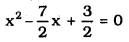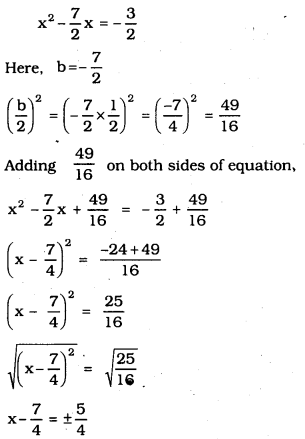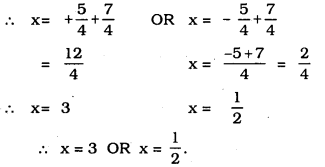(ii) 2x2 + x – 4 = 0
Dividing all terms by 2,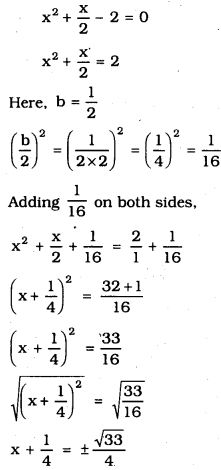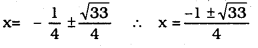(iii) 4x2 + 43–√x + 3 = 0
Dividing all terms by 4,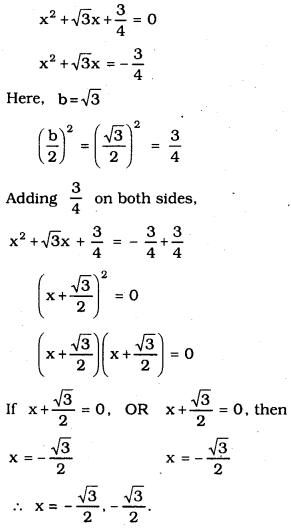(iv) 2x2 + x + 4 = 0
Dividing all terms of the equation by 2,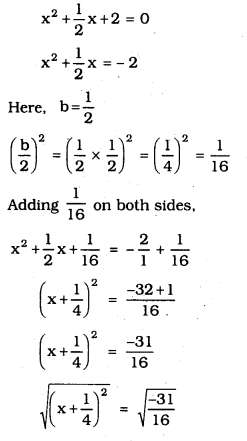Here ‘x’ has no fixed value because −31−−−−√ is not a square root.

Quadratic Equation Exercise 10.3 Question 2.
Find the roots of the quadratic equations given in Q.1 above by applying the quadratic formula.
(i) 2x2 – 7x + 3 = 0.
(ii) 2x2 + x – 4 = 0
(iii) 4x2 + 43–√x + 3 = 0
(iv) 2x2 + x + 4 = 0
Solution:
(i) 2x2 – 7x + 3 = 0.
Here, a = 2, b = -7, c = 3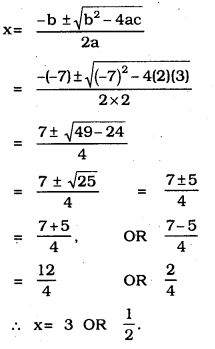(ii) 2x2 + x – 4 = 0
Here, a = 2, b = 1, c = 4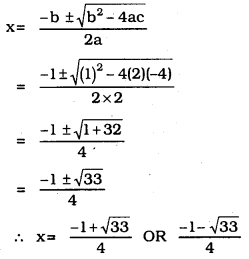(iii) 4x2 + 43–√x + 3 = 0
Here, a = 4, b = 43–√, c = 3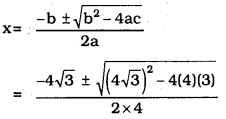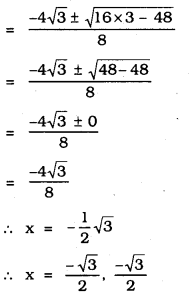(iv) 2x2 + x + 4 = 0
Here, a = 2, b = 1, c = 4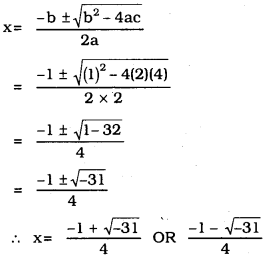10th Maths Quadratic Equations Exercise 10.3 Question 3.
Find the roots of the following equations: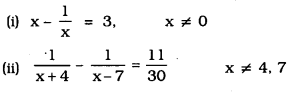Solution: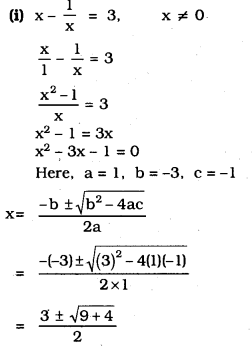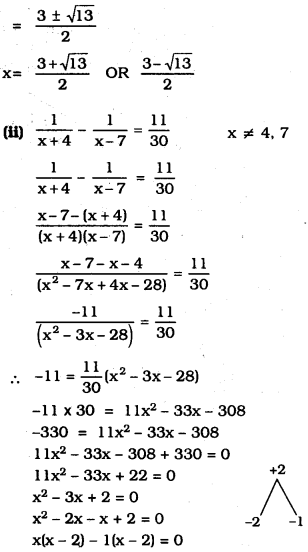(x – 2) (x – 1) = 0
If x – 2 = 0, then x = 2
If x – 1 = 0, then x = 1
∴ x = 2 OR x = 1.

Quadratic Equation Class 10 Exercise 10.3 Question 4.
The sum of the reciprocals of Rehman’s ages, (in years) 3 years ago and 5 years from now is 13. Find his present age.
Solution:
Let the present age of Rehman be ‘x’ years.
After 5 years his age will be (x + 5)
3 years back his age was (x – 3)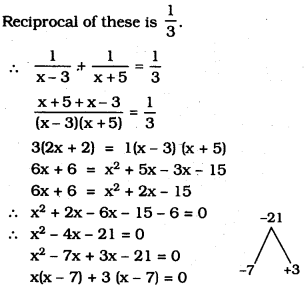(x – 7) (x + 3) = 0
If x – 7 = 0, then x = 7
If x + 3 = 0, then x = -3
∴ x = 7 OR x = -3
∴ Present age of Rehman is 7 years.

KSEEB Solutions For Class 10 Maths Quadratic Equation Question 5.
In a class test, the sum of Shefali’s marks in Mathematics and English is 30. Had she got 2 marks more in Mathematics and 3 marks less in English, the product of their marks would have been 210. Find her marks in the two subjects.
Solution:
Let Shefali’s marks in mathematics is Y and marks in English is (30 – x)
Shefali’s gets 2 more marks in mathematics then x + 2 and 3 marks less in English is (30 – x – 3)
Product of Shefali’s marks = 210
(x + 2) (30 – x – 3) = 210
(x + 2) (27 – x) = 210
27x + 54 – x2 – 2x = 210
– x2 + 25x + 54 = 210
x2 – 25x + 210 – 54 = 0
x2 – 25x + 156 = 0
x2 – 13x – 12x + 156 = 0
x(x – 13) – 12 (x – 13) = 0
(x – 13) (x – 12) = 0
x – 13 = 0 (or) x – 12 = 0
x = 13 (or) x = 12
Therefore, Shefali’s marks in Mathematics =13
Marks in English = 30 – 13 = 17 (or) Shefali’s marks in Mathematics = 12 then.
Marks in English = 30 – 12 = 18

The diagonal of a rectangular field is 60 metres more than the shorter side. If the longer side is 30 metres more than the shorter side, find the sides of the field.
Solution: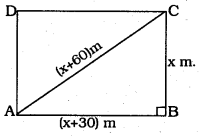Let shorter side of rectangle ABCD be x metre,
Then, longer side of rectangle ABCD,
AB = (x + 30) m.
Diagonal AC = (x + 60) m.
In ⊥∆ABC, ∠B = 90°.
As per Pythagoras theorem,
AB2 + BC2 = AC2
(x + 30)2 + (x)2 = (x + 60)2
x2 + 60x + 900 + x2 = x2 + 120x + 3600
2x2 + 60x + 900 = x2 + 120x + 3600
2x2 – x2 + 60x – 120x + 900 – 3600 = 0
x2 – 60x – 2700 = 0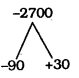x2 – 90x + 30x – 2700 = 0
x(x – 90) + 30 (x – 90) = 0
(x – 90) (x + 30) = 0
If x – 90 = 0, then x = 90
If x + 30 = 0, then x = -30
Shorter side of rectangle, BC = x = 90 m.
Longer side of rectangle, AB = x + 30 = 90 + 30= 120 m.
Diagonal of rectangle, AC = x + 60 = 90 + 60 = 150 m.

Quadratic Equations Exercise 10.3 Question 7.
The difference of squares of two numbers is 180. The square of the smellier number is 8 times the larger number. Find the two numbers.
Solution:
Let the small number be ‘x’.
The Square of the smaller number is 8 times the larger number.
∴ x2 = 8 × Larger number.
∴ Larger number = 18 x2.
The difference between these numbers is 180.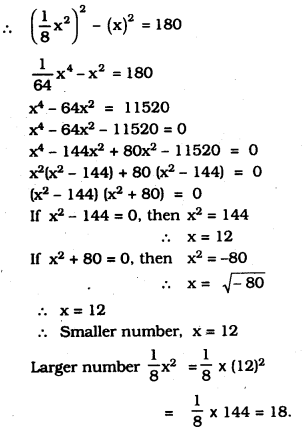∴ Smaller number is 12,
Larger number is 18.

Ex 10.3 Quadratic Equation Question 8.
A train travels 360 km at a uniform speed. If the speed had been 5 km/h more, it would have taken 1 hour less for the same journey. Find the speed of the train.
Solution:
Let initial speed of the train be x km/h,
Then, time taken to cover 360 km
If the speed had been 5 km/h more,
360x Hr.
then time taken to cover is 360x+5 Hr.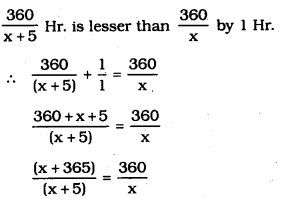x(x + 365) = 360 (x + 5)
x2 + 365x = 360x + 1800
x2 + 365x – 360x – 1800 = 0
x2 + 5x – 1800 = 0
x2 + 45x – 40x – 1800 = 0
x(x + 45) – 40 (x + 45) = 0
(x + 45) (x – 40) = 0
If x + 45 = 0, then x = – 45
If x – 40 = 0, then x = 40
∴ Initial speed of a train is 40 km/hr.

Quadratic Equation 10.3 Exercise Question 9.
Two water taps together can fill a tank in 938 hours. The tap of a larger diameter takes 10 hours less than the smaller one to fill the tank separately. Find the time in which each tap can separately fill the tank. .
Solution:
Let the time required to fill up the tank for tap having a larger diameter be ‘x’ Hr. For ‘x’ hour part of the tank filling is 1.
For 938 hr. part of the tank filling ………..?
i.e., 75×18x part
Time required for smaller diameter is = (x – 10)Hr.
For (x – 10)Hr part of the tank, filling is 1
For 938 Hr. part of the tank is …………?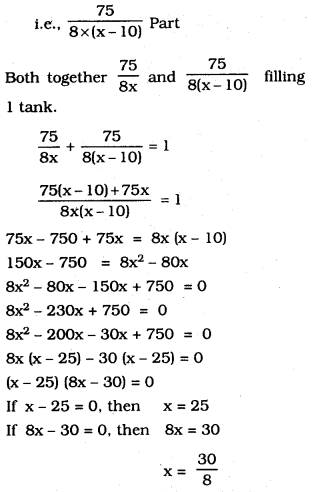∴Time required for larger diameter, x = 25 Hr.
∴ Time required for smaller diameter = x – 10 = 15 Hr.

Quadratic Equations Ex 10.3 Question 10.
An express train takes 1 hour less than a passenger train to travel 132 km between Mysore and Bangalore (without taking into consideration the time they stop at intermediate stations). If the average speed of the express train is 11km/h more than that of the passenger train, find the average speed of the two trains.
Solution:
Let Average speed of passenger train be = x km/h.
. But Average speed of Express train be = (x + 11) km/h.
Time required for Passenger:
For travelling x km time required is 1 Hr
For travelling 132 km time required …….?
132x Hr.
Time required for Express train :
For travelling (x + 11) km is 1 Hr.
For travelling 132 km …………?
i.e., 132(x+11) Hr
Express train required 1 Hr. less comparing to Passenger train. .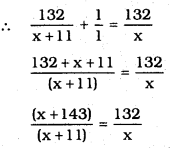x(x + 143) = 132 (x+ 11)
x2 + 143x = 132x + 1452
x2 + 143x – 132 x – 1452 = 0
x2 + 11x – 1452 = 0
x2 + 44x – 33x – 1452 = 0
x(x + 44) – 33(x + 44) = 0
(x + 44) (x – 33) = 0
If x + 44 = 0, then x = -44
If x – 33 = 0, then x = 33
∴ Average speed of passenger train is 33 km/hr.
∴ Average speed of Express train = x + 11 = 33 + 11 = 44 km/hr.

All Chapter KSEEB Solutions For Class 10 Maths

—————————————————————————–

All Subject KSEEB Solutions For Class 10

*************************************************

I think you got complete solutions for this chapter. If You have any queries regarding this chapter, please comment on the below section our subject teacher will answer you. We tried our best to give complete solutions so you got good marks in your exam.

If these solutions have helped you, you can also share kseebsolutionsfor.com to your friends.

Best of Luck!!